## Section3.9Vectors Bootcamp

### Subsection3.9.4Miscellaneous

In a triangle $\triangle ABC \text{,}$ let $a,\, b,$ and $c$ denote the lengths of the sides, and C denote the angle between sides $a$ and $b\text{.}$ Use vector concepts to prove the following relation.

\begin{equation*} c^2 = a^2 + b^2 -2\, a\, b\, \cos\, C. \end{equation*}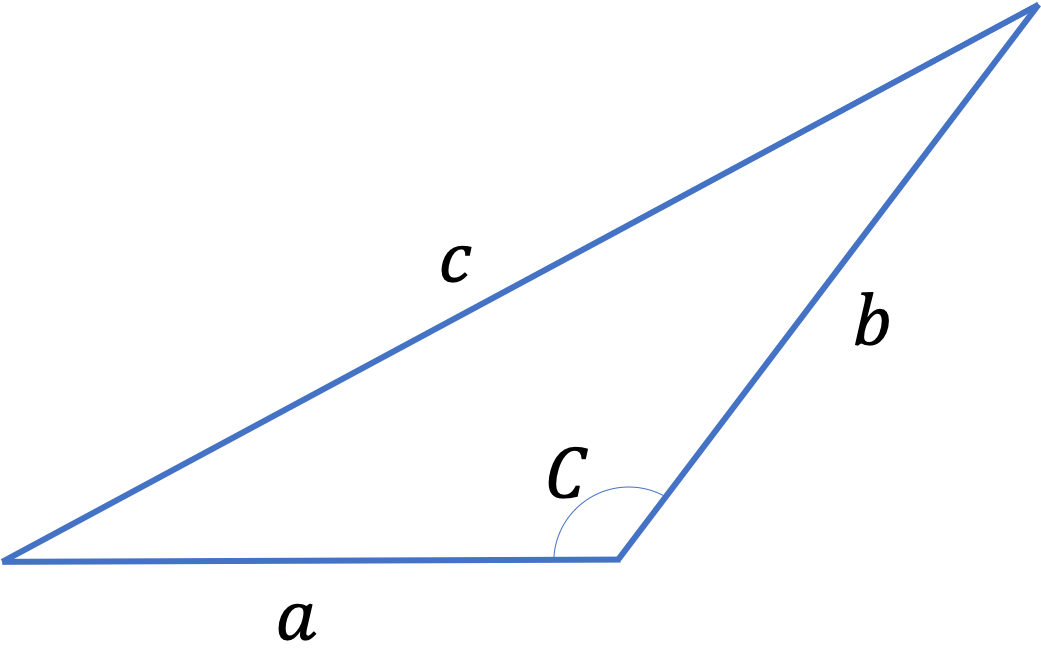Hint

Use a triangle of vectors oriented such that $\vec c = \vec a + \vec b \text{.}$

In the problem statement.

Solution

Consider a triangle of vectors along the sides of the triangle as shown in Figure 3.9.36. From this figure, we get the following vector relation.

\begin{equation*} \vec c = \vec a + \vec b. \end{equation*}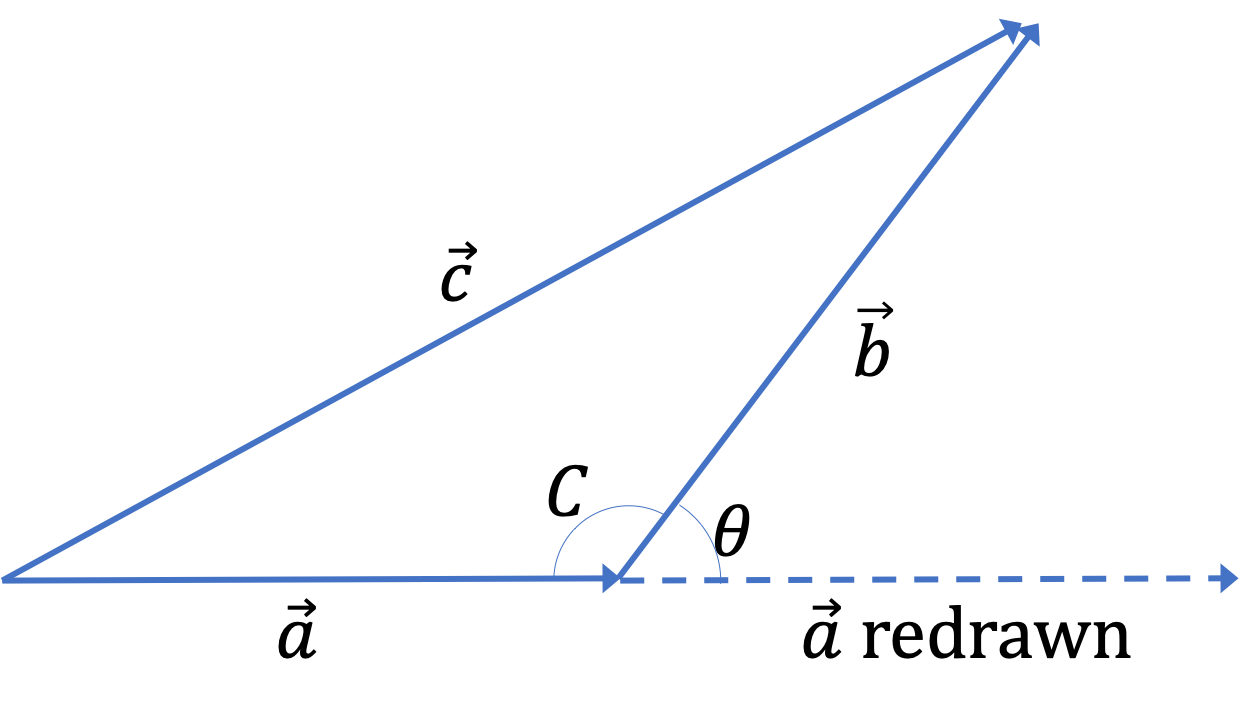Figure 3.9.36. The arrows on the sides show that $\vec c = \vec a + \vec b\text{.}$ The angle $\theta$ between the vectors $\vec a$ and $\vec b$ is the angle when the tails of both of these vectors are at the same place, as obtained by redrawing vector $\vec a$ shown. the redrawing shows that $\theta = 180^{\circ} -C\text{.}$

Now, taking the dot product of each side with itself, we get

\begin{equation} \vec c \cdot \vec c = \vec a \cdot \vec a + \vec b \cdot \vec b + 2\vec a \cdot \vec b.\label{eq-Prove-the-Law-of-Cosine}\tag{3.9.1} \end{equation}

We can rewrite the last term in terms of the angle between vectors $\vec a$ and $\vec b \text{,}$ which is just $180^{\circ} -C\text{.}$ Therefore,

\begin{equation*} \vec a \cdot \vec b = a\, b\, \cos(180^{\circ} -C) = -a\, b\, \cos\,C. \end{equation*}

Using this in Eq. (3.9.1) gives the result we seek.

\begin{equation*} c^2 = a^2 + b^2 -2\, a\, b\, \cos\, C. \end{equation*}

In a triangle $\triangle ABC \text{,}$ let $a,\, b,$ and $c$ denote the lengths of the sides, and A, B, C denote the angles opposite to the corresponding sides as shown in the figure. Use vector concepts to prove the following relations.

\begin{equation*} \dfrac{\sin\, A}{a} = \dfrac{\sin\, B}{b} = \dfrac{\sin\, C}{c}. \end{equation*}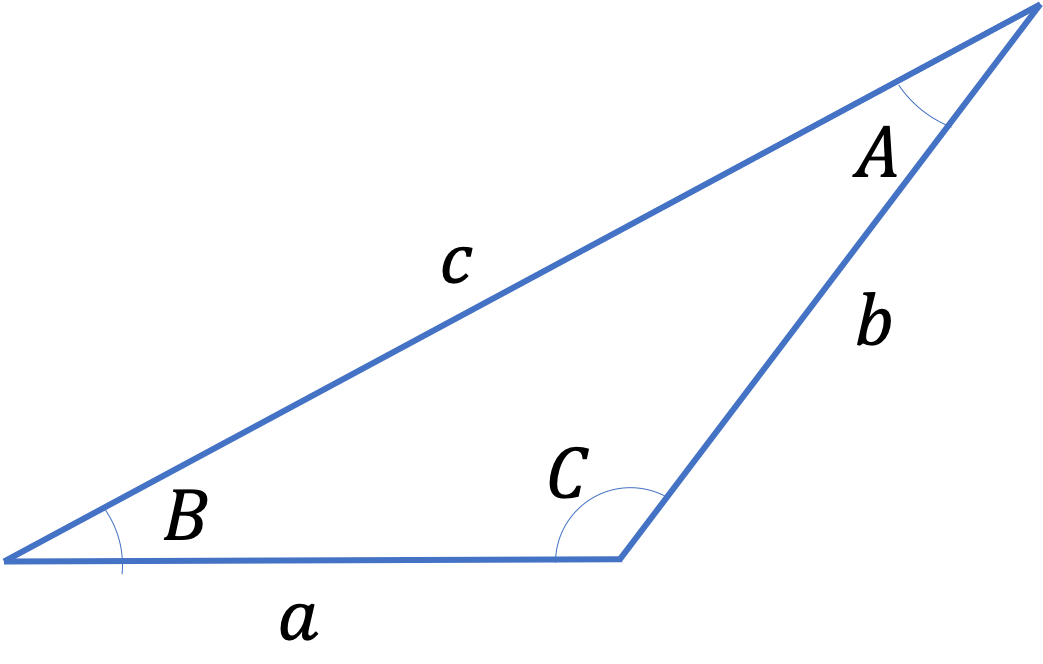Hint

Examine height of the triangle.

In the problem statement.

Solution

Look at the construction in the figure below, where height from the tip with angle $\langle A$ to the the (extended) base on the other side is shown.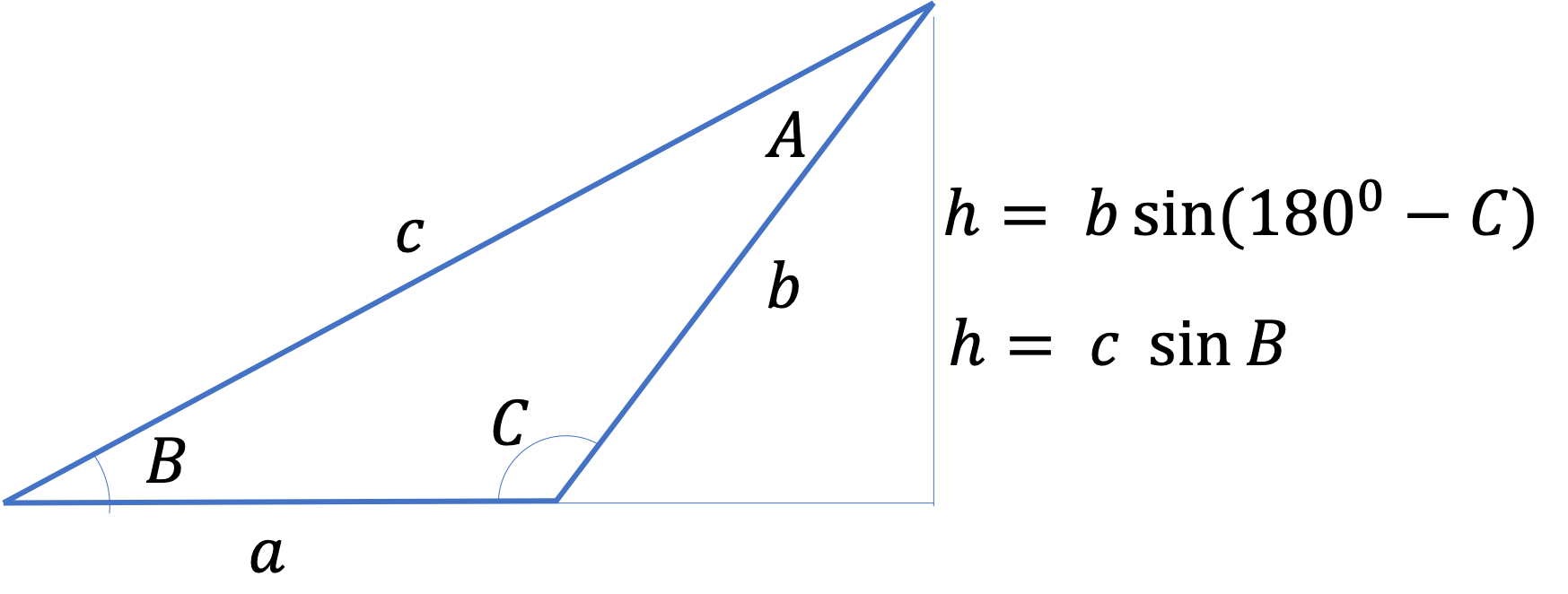Since

\begin{equation*} \sin\left( 180^{\circ} -C \right) = \sin\, C, \end{equation*}

equating the two expressions for the height gives

\begin{equation*} c\, \sin\, B = b\, \sin\, C. \end{equation*}

Therefore,

\begin{equation*} \dfrac{\sin\, B}{b} = \dfrac{\sin\, C}{a}. \end{equation*}

Similar construction from the tip at angle $\langle C$ will show

\begin{equation*} \dfrac{\sin\, A}{a} = \dfrac{\sin\, B}{b}. \end{equation*}

The two equations taken together prove the statement of the law of sines.

Suppose you and your friend are on a large ship. Suppose the ship has a velocity of $3$ m/s pointed towards the positive $x$ axis as seen from the shore. Suppose your friend is moving with respect to you (i.e., the ship) at a velocity of $4$ m/s towards the positive $y$ axis. What is your friend's velocity vector with respect to the shore. Find both component, and the magnitude and direction forms.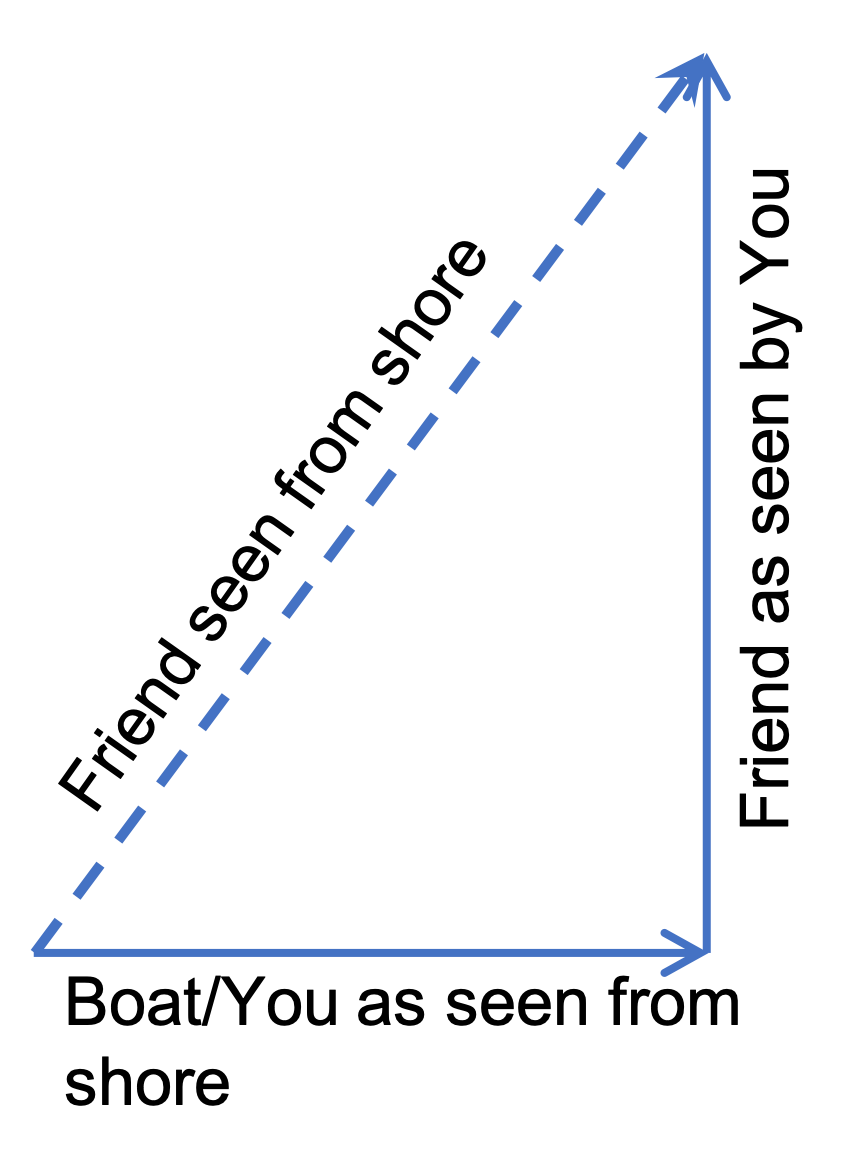Note that a good notation will help. For instance, we can label each velocity with two subscripts, one for the object and the second the object with respect to which we have that velocity. Thus, let $\vec v_{SG}$ will be velocity vector of the ship with respect to the ground. This is given in componen form, $\vec v_{SG} = (3 \text{ m/s}, 0)$ in the $xy$-plane. Similarly, $\vec v_{FS} = (0, 4 \text{ m/s})\text{.}$ And, we want $\vec v_{FG} \text{.}$

Hint

Use $\vec v_{FG} = \vec v_{FS} + \vec v_{SG}\text{.}$

$5 \text{ m/s at } 53.1^{\circ} \text{ counterclockwise from } +x \text{ axis}.$

Solution

The relation between velocities, as seen in the figure provided, can be formally written as

\begin{equation*} \vec v_{FG} = \vec v_{FS} + \vec v_{SG}. \end{equation*}

We can use the vector addition of components to obtain the components of $\vec v_{FG} \text{.}$

\begin{align*} \vec v_{FG} \amp = \vec v_{FS} + \vec v_{SG}\\ \amp = (0, 4 \text{ m/s}) + (3 \text{ m/s}, 0)\\ \amp = (3 \text{ m/s}, 4 \text{ m/s}) \end{align*}

From this we can find the magnitude and angle $\theta \text{.}$

\begin{align*} \amp \text{Magnitude } = \sqrt{3^2 + 4^2} = 5\text{ m/s}.\\ \amp \theta = \tan^{-1} \dfrac{4}{3} = 53.1^{\circ} \end{align*}

Since the vector $(3 \text{ m/s}, 4 \text{ m/s})$ is in the first qudrant, the angle we foound is the counterclockwise angle from the $+x$ axis.

In order for you to cross a river exactly on the opposite side from one shore, you have to point the boat slightly up stream. This happens because your velocity with respect to shore is different from your velocity with respect to the river.

Let $\vec v_{BS}$ be the velocity of the boat (B) with respect to the shore (S), $\vec v_{BR}$ be the velocity of the boat (B) with respect to the river (R), and $\vec v_{RS}$ be the velocity of the river stream (R) with respect to the shore (S). These vectors form a triangle as shown in the figure below. Notice that vectors $\vec v_{BR}$ and $\vec v_{RS}$ must add up to the vector $\vec v_{BS} \text{.}$

\begin{equation} \vec v_{BS} = \vec v_{BR} + \vec v_{RS}.\label{eq-crossing-river}\tag{3.9.2} \end{equation}Now, the question. In order to cross the river directly on the other side, suppose you need to row the boat at speed $5\text{ m/s}$ at $\theta=30^{\circ}$ to directly across direction, which is the velocity of the boat with respect to the river. (a) What is the speed of the river? (b) If the river is $100\text{ m}$ wide, how long would it take to cross the rivew?

Hint

Express velocities in coordinate form and use Eq. (3.9.2).

(a) $2.5\text{ m/s}\text{,}$ (b) $23.1\text{ s}\text{.}$

Solution

(a) Let's use simpler notation in our calculations. Let $v$ denote the speed of the river with respect to the shore. Let $u$ be the speed of the boat with respect to the shore. Let $x$ axis be in the direction of the river flow and $y$ axis directly across. Then, Eq. (3.9.2), written using components form of the vectors is (suppressing the units):

\begin{equation*} (0, u) = (-5\sin 30^{\circ}, 5\cos 30^{\circ}) + (v, 0). \end{equation*}

This gives us two equations, one along the $x$ axis and the other along the $y$ axis.

\begin{gather*} 0 = -5\sin 30^{\circ} + v \\ u = 5\cos 30^{\circ} + 0 \end{gather*}

This gives $u = 4.33\text{ m/s}$ and $v = 2.5 \text{ m/s}\text{.}$ We will use $u$ in part (b). The river is flowing at speed $v = 2.5\text{ m/s} \text{.}$

(b) The boat moves at speed $u = 4.33$ m/s across the river which is $d = 100\text{ m} \text{.}$ Therefore, it will take

\begin{equation*} t = \dfrac{d}{u} = \dfrac{100\text{ m}}{4.33 \text{ m/s}} = 23.1\text{ s}. \end{equation*}

Two vectors $\vec A$ and $\vec B$ are defined as $\vec A=a\hat i$ and $\vec B = a\left(\cos\,\omega t\,\hat i + \sin\,\omega t\,\hat j \right)\text{,}$ where $a$ is a constant and $\omega = \pi/6\text{ rad s}^{-1}\text{.}$

If $\left| \vec A + \vec B\right| = \sqrt{3} \left| \vec A - \vec B\right|$ at time $t=\tau$ for the first time, find then value of $\tau$ in seconds. (Adpated from Indian JEE Advanced, 2018)

Hint

Use definition of square of amplitude of a vector.

$2.0\text{ sec}\text{.}$

Solution

This question can be answered by performing the algebra on the given condition.

\begin{align*} \amp \vec A = a\hat i,\ \ \vec B = a\left(\cos\,\omega t\,\hat i + \sin\,\omega t\,\hat j \right)\\ \amp \vec A + \vec B= (a + a \cos\,\omega t)\,\hat i + a \sin\,\omega t\,\hat j \\ \amp \vec A - \vec B= (a - a \cos\,\omega t)\,\hat i - a \sin\,\omega t\,\hat j \end{align*}

Therefore, square of $\left| \vec A + \vec B\right| = \sqrt{3} \left| \vec A - \vec B\right|$ at $t=\tau$ becomes

\begin{equation*} (a + a \cos\,\omega \tau)^2 + a^2 \sin^2\omega \tau = (\sqrt{3})^2\left[ (a - a \cos\,\omega \tau)^2 + a^2 \sin^2\omega \tau\right]. \end{equation*}

We can cancel out $a^2$ from every term, expand $(1+\cos x)^2$ and use $\sin^2 x + \cos^2 x = 1\text{,}$ where $x=\omega \tau\text{.}$

\begin{equation*} 1 + \cos\,\omega\tau = 3 ( 1 - \cos\,\omega\tau). \end{equation*}

This yields

\begin{equation*} \cos\,\omega\tau =\frac{1}{2}. \end{equation*}

Since $\cos^{-1}\left(\frac{1}{2} \right) = \frac{\pi}{3}\text{ rad}\text{,}$ we have

\begin{align*} \tau \amp = \frac{6}{\pi}\times \frac{\pi}{3}= 2.0\text{ sec}. \end{align*}

Often, we are interested in how much of a vector is along some given unit vector and along some direction perpendicular to that unit vector. Let $\hat u$ be a given unit vector. Show the following relation holds for an arbitrary vector $\vec A\text{.}$

\begin{equation*} \vec A = \vec A_\parallel + \vec A_\perp, \end{equation*}

where the parallel and perpendicular components of $\vec A$ are

\begin{align*} \amp \vec A_\parallel = (\hat u \cdot \vec A)\, \hat u,\ \ \ \vec A_\perp = (\hat u \times \vec A)\times \hat u. \end{align*}

Prove the following identities for two arbitrary vectors $\vec A$ and $\vec B\text{.}$

\begin{align} \amp \vec A \cdot \vec B = \vec B \cdot \vec A\tag{3.9.3}\\ \amp \vec A \times \vec B = - \vec B \times \vec A\tag{3.9.4} \end{align}
Solution

No solution provided.

Prove the following identitiy for three arbitrary vectors $\vec A\text{,}$ $\vec B\text{,}$ and $\vec C\text{,}$ called the cab-bac equation.

\begin{equation*} \vec A \times (\vec B \times \vec C)= (\vec C\cdot \vec A) \vec B - (\vec B\cdot \vec A)\vec C \end{equation*}

Maybe you want to show that $x$ component of each side comes out the same.

Solution

No solution provided.

A parallelopiped can be described by stating three vectors along its edges as shown in Figure 3.9.45. Just as area of the base here could be obtained from the magnitude of vector product $|\vec B \times \vec C|\text{,}$ the volume of the parallelopiped can be obtained by a scalar triple prroduct.

\begin{equation*} \vec A \cdot (\vec B \times \vec C). \end{equation*}

Prove that volume is indeed given by this formula.

Also show that you get the same answer by cyclically rotating the vector names, e.g.,

\begin{equation*} \vec A \cdot (\vec B \times \vec C) = \vec B \cdot (\vec C \times \vec A) = \vec C \cdot (\vec A \times \vec B). \end{equation*}
Solution

No solution provided.

If the diagonals of a parallelogram are equal, then the sides must be perpendicular to each other.

Hint

Place two vectors $\vec A$ and $\vec A$ on two adjecent sides. Then, notice that the diagonals are $\vec A + \vec B$ and $\vec A - \vec B\text{.}$ Use dot product between them to prove the result.

Solution

No solution provided.